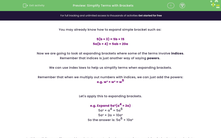# Simplify Terms with Brackets

In this worksheet, students will expand and simplify brackets, applying index laws when working with powers.Key stage:  KS 4

Year:  GCSE

GCSE Subjects:   Maths

GCSE Boards:   Pearson Edexcel, OCR, Eduqas, AQA,

Curriculum topic:   Algebra

Curriculum subtopic:   Notation, Vocabulary and Manipulation Algebraic Expressions

Popular topics:   Algebra worksheets

Difficulty level:#### Worksheet Overview

You may already know how to expand simple bracket such as:

5(b + 3) = 5b + 15

5a(b + 4) = 5ab + 20a

Now we are going to look at expanding brackets where some of the terms involve indices.

Remember that indices is just another way of saying powers

We can use index laws to help us simplify terms when expanding brackets.

Remember that when we multiply out numbers with indices, we can just add the powers:

e.g. w³ × w² = w5

Let's apply this to expanding brackets.

e.g. Expand 5a²(a4 + 2a)

5a² × a4 = 5a6

5a² × 2a = 10a³

So the answer is: 5a6 + 10a³

In this activity, we will expand brackets to create simpler expressions, applying index laws to calculate successfully when working with powers.

### What is EdPlace?

We're your National Curriculum aligned online education content provider helping each child succeed in English, maths and science from year 1 to GCSE. With an EdPlace account you’ll be able to track and measure progress, helping each child achieve their best. We build confidence and attainment by personalising each child’s learning at a level that suits them.

Get started